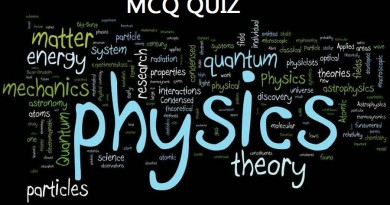Latest SSC jobs   »   Physics Quiz on “RESISTANCE” Based on...

# Physics Quiz on “RESISTANCE” Based on NCERT for SSC 20161. Resistance is:
A. the opposition to current flow accompanied by the dissipation of heat
B. symbolized by R, measured in ohms metre, and directly proportional to conductance
C. directly proportional to current and voltage
D. represented by the flow of fluid in the fluid circuit

2. With Ohm’s law, if voltage increases and resistance stays the same:
A. current remains the same
B. power decreases
C. current increases
D. resistance decreases
3. Ohm’s law is not:
A. V = IR
B. I = V/R
C. R = IV
D. R = V/I

4. With Ohm’s law:
A. current is inversely proportional to resistance
B. resistance is directly proportional to voltage
C. voltage is indirectly proportional to power
D. current is directly proportional to resistance
5. How do fixed resistors usually fail?
A. slowly over time
B. by increasing their value
C. by becoming an open circuit
D. by increasing their value and becoming an open circuit
6. What happens to current and resistance if the voltage doubles?
A. Current doubles and resistance doubles.
B. Current doubles and resistance is halved.
C. Current remains the same and resistance doubles.
D. Current doubles and resistance remains the same.
7. If a variable resistor’s resistance varies in a non-uniform manner as the shaft is moved, it is considered to be:
A. linear
B. defective
C. not wirewound
D. tapered
8. How many connections does a rheostat have?
A. 1
B. 2
C. 3
D. 4
Ans. B

9. The resistivity of copper is:
A. 9.9 Ωm
B. 1.68 Ωm
C. 16.7 Ω m
D. 1.80 Ωm
10. What are the parts of a rheostat?
A. center tape and wiper
B. solenoid and armature
C. contact and wire wound
D. wiper and resistor track